# High School Math : How to find the perimeter of a rectangle

## Example Questions

### Example Question #1 : How To Find The Perimeter Of A Rectangle

A rectangle has a width of 2x. If the length is five more than 150% of the width, what is the perimeter of the rectangle?

10(x + 1)

6x2 + 5

5x + 10

5x + 5

6x2 + 10x

10(x + 1)

Explanation:

Given that w = 2x and l = 1.5w + 5, a substitution will show that l = 1.5(2x) + 5 = 3x + 5.

P = 2w + 2l = 2(2x) + 2(3x + 5) = 4x + 6x + 10 = 10x + 10 = 10(x + 1)

### Example Question #1 : How To Find The Perimeter Of A Rectangle

What is the perimeter of the below rectangle in simplest radical form?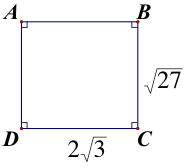10√3

4√3 + 2√27

7√27

5√3

10√3

Explanation:

The perimeter of a figure is the sum of the lengths of all of its sides. The perimeter of this figure is √27 + 2√3 + √27 + 2√3. But, √27 = √9√3 = 3√3 . Now all of the sides have the same number underneath of the radical symbol (i.e. the same radicand) and so the coefficients of each radical can be added together. The result is that the perimeter is equal to 10√3.

### Example Question #1 : How To Find The Perimeter Of A Rectangle

A rectangle has an area of 56 square feet, and a width of 4 feet. What is the perimeter, in feet, of the rectangle?

120
14
28
36
30
Explanation:

Divide the area of the rectangle by the width in order to find the length of 14 feet. The perimeter is the sum of the side lengths, which in this case is 14 feet + 4 feet +14 feet + 4 feet, or 36 feet.

### Example Question #1 : How To Find The Perimeter Of A Rectangle

The length of a rectangle is 3 more inches than its width.  The area of the rectangle is 40 in2.  What is the perimeter of the rectangle?

None of the answers are correct

34 in.

40 in.

26 in.

18 in.

26 in.

Explanation:

Area of rectangle:  A = lw

Perimeter of rectangle:  P = 2l + 2w

w = width and l = w + 3

So A = w(w + 3) = 40 therefore w2 + 3w – 40 = 0

Factor the quadratic equation and set each factor to 0 and solve.

w2 + 3w – 40 = (w – 5)(w + 8) = 0 so w = 5 or w = -8.

The only answer that makes sense is 5.  You cannot have a negative value for a length.

Therefore, w = 5 and l = 8, so P = 2l + 2w = 2(8) + 2(5) = 26 in.

### Example Question #3 : How To Find The Perimeter Of A Rectangle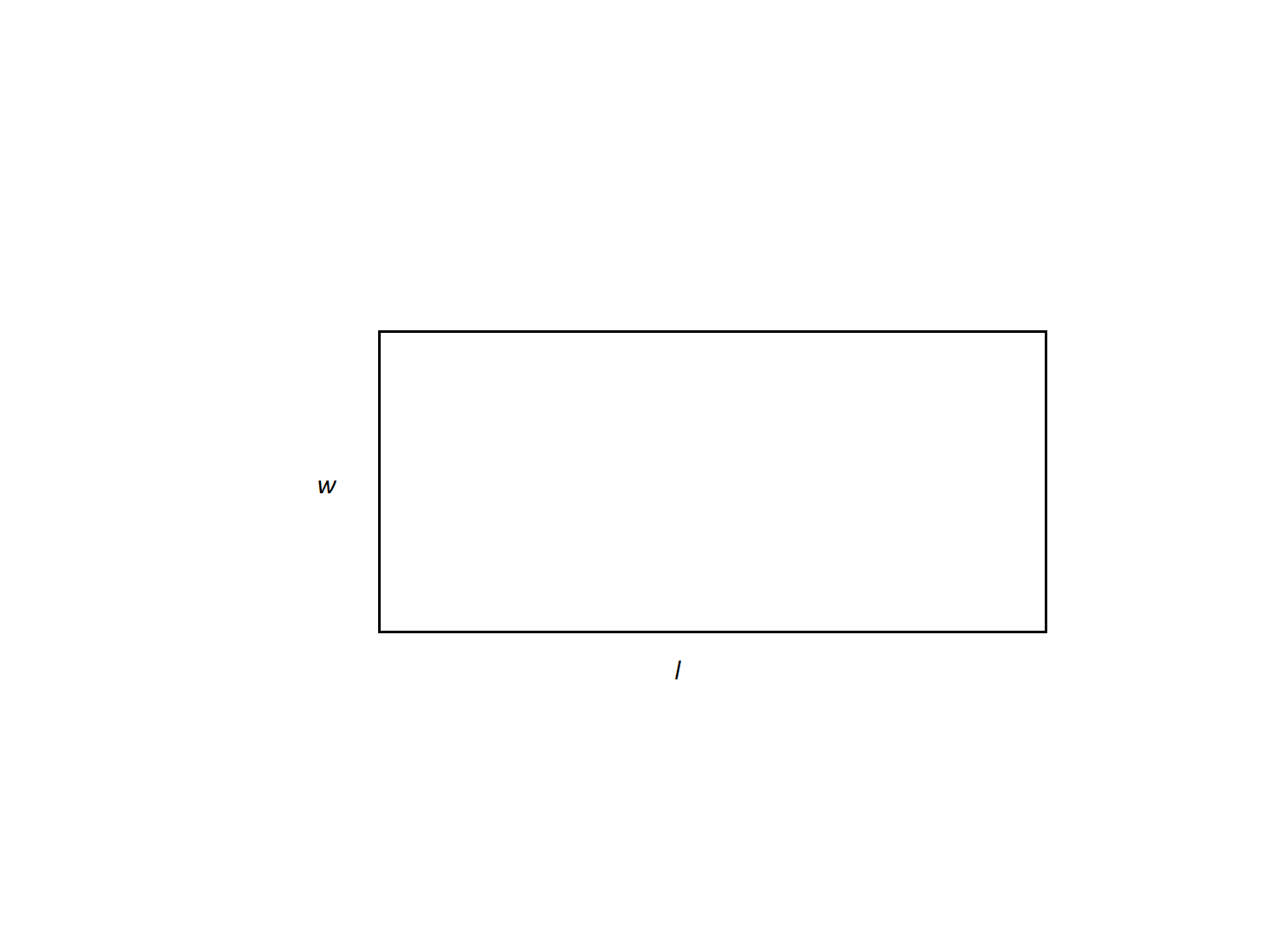The length of a rectangle is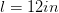and the width is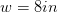. What is its perimeter?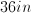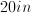Not enough information to solve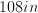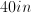Explanation:

Perimeter of a quadrilateral is found by adding up the lengths of its sides. The formula for the perimeter of a rectangle is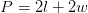.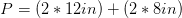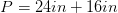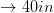### Example Question #4 : How To Find The Perimeter Of A Rectangle

Kayla took 25 minutes to walk around a rectangular city block. If the block's width is 1/4 the size of the length, how long would it take to walk along one length?

10 minutes

2.5 minutes

7 minutes

8 minutes

10 minutes

Explanation:

Leaving the width to be x, the length is 4x. The total perimeter is 4x + 4x + x + = 10x.

We divide 25 by 10 to get 2.5, the time required to walk the width.  Therefore the time required to walk the length is (4)(2.5) = 10.

### Example Question #9 : How To Find The Perimeter Of A Rectangle

Robert is designing a rectangular garden. He wants the area of the garden to be 9 square meters. If the length of the lot is going to be three meters less than twice the width, what will the perimeter of the lot be in meters?

3

6

10

1.5

12

12

Explanation:

Let l be the length of the garden and w be the width.

By the specifications of the problem, l = 2w-3.

Plug this in for length in the area formula:

A = l  x w = (2w - 3) x w = 9

Solve for the width:

2w²- 3w - 9 =0

(2w + 3)(w - 3) = 0

w is either 3 or -3/2, but we can't have a negative width, so w = 3.

If w = 3, then length = 2(3) - 3 = 3.

Now plug the width and length into the formula for perimeter:

P = 2 l + 2w = 2(3) + 2(3) = 12

### Example Question #1 : Rectangles

A rectangular garden has an area of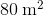. Its length ismeters longer than its width. How much fencing is needed to enclose the garden?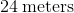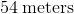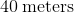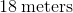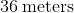Explanation:

We define the variables as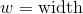and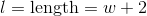.

We substitute these values into the equation for the area of a rectangle and get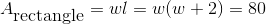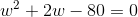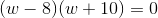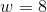or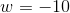Lengths cannot be negative, so the only correct answer is. If, then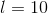Therefore,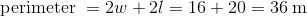.